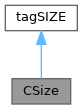ReactOS 0.4.15-dev-6675-gcbc63d8
CSize Class Reference

`#include <atltypes.h>`

Inheritance diagram for CSize:[legend]
Collaboration diagram for CSize:[legend]

## Public Member Functions

CSize () throw ()

CSize (int initCX, int initCY) throw ()

CSize (SIZE initSize) throw ()

CSize (POINT initPt) throw ()

CSize (DWORD dwSize) throw ()

BOOL operator== (SIZE size) const throw ()

BOOL operator!= (SIZE size) const throw ()

void operator+= (SIZE size) throw ()

void operator-= (SIZE size) throw ()

CSize operator+ (SIZE size) const throw ()

CPoint operator+ (POINT point) const throw ()

CRect operator+ (const RECT *lpRect) const throw ()

CSize operator- (SIZE size) const throw ()

CPoint operator- (POINT point) const throw ()

CRect operator- (const RECT *lpRect) const throw ()

CSize operator- () const throw ()Public Attributes inherited from tagSIZE
LONG cx

LONG cy

## Detailed Description

Definition at line 132 of file atltypes.h.

## ◆ CSize() [1/5]

 CSize::CSize ( ) throw ( )
inline

Definition at line 135 of file atltypes.h.

136 {
137 cx = cy = 0;
138 }
LONG cx
Definition: windef.h:334
LONG cy
Definition: windef.h:335

Referenced by operator+(), and operator-().

## ◆ CSize() [2/5]

 CSize::CSize ( int initCX, int initCY ) throw ( )
inline

Definition at line 140 of file atltypes.h.

141 {
142 cx = initCX;
143 cy = initCY;
144 }

## ◆ CSize() [3/5]

 CSize::CSize ( SIZE initSize ) throw ( )
inline

Definition at line 146 of file atltypes.h.

147 {
148 *((SIZE*)this) = initSize;
149 }

## ◆ CSize() [4/5]

 CSize::CSize ( POINT initPt ) throw ( )
inline

Definition at line 151 of file atltypes.h.

152 {
153 *((POINT*)this) = initPt;
154 }

## ◆ CSize() [5/5]

 CSize::CSize ( DWORD dwSize ) throw ( )
inline

Definition at line 156 of file atltypes.h.

157 {
158 cx = LOWORD(dwSize);
159 cy = HIWORD(dwSize);
160 }
PSDBQUERYRESULT_VISTA PVOID DWORD * dwSize
Definition: env.c:56
#define LOWORD(l)
Definition: pedump.c:82
#define HIWORD(l)
Definition: typedefs.h:247

## ◆ operator!=()

 BOOL CSize::operator!= ( SIZE size ) const throw ( )
inline

Definition at line 167 of file atltypes.h.

168 {
169 return !(*this == size);
170 }
GLsizeiptr size
Definition: glext.h:5919

## ◆ operator+() [1/3]

 CRect CSize::operator+ ( const RECT * lpRect ) const throw ( )
inline

Definition at line 556 of file atltypes.h.

557{
558 CRect r(lpRect);
559 r += *this;
560 return r;
561}
GLdouble GLdouble GLdouble r
Definition: gl.h:2055

## ◆ operator+() [2/3]

 CPoint CSize::operator+ ( POINT point ) const throw ( )
inline

Definition at line 189 of file atltypes.h.

190 {
191 return CPoint(cx + point.x, cy + point.y);
192 }
POINTL point
Definition: edittest.c:50
LONG y
Definition: windef.h:330
LONG x
Definition: windef.h:329

## ◆ operator+() [3/3]

 CSize CSize::operator+ ( SIZE size ) const throw ( )
inline

Definition at line 184 of file atltypes.h.

185 {
186 return CSize(cx + size.cx, cy + size.cy);
187 }
CSize()
Definition: atltypes.h:135

## ◆ operator+=()

 void CSize::operator+= ( SIZE size ) throw ( )
inline

Definition at line 172 of file atltypes.h.

173 {
174 cx += size.cx;
175 cy += size.cy;
176 }

## ◆ operator-() [1/4]

 CSize CSize::operator- ( ) const throw ( )
inline

Definition at line 208 of file atltypes.h.

209 {
210 return CSize(-cx, -cy);
211 }

## ◆ operator-() [2/4]

 CRect CSize::operator- ( const RECT * lpRect ) const throw ( )
inline

Definition at line 563 of file atltypes.h.

564{
565 CRect r(lpRect);
566 r -= *this;
567 return r;
568}

## ◆ operator-() [3/4]

 CPoint CSize::operator- ( POINT point ) const throw ( )
inline

Definition at line 201 of file atltypes.h.

202 {
203 return CPoint(cx - point.x, cy - point.y);
204 }

## ◆ operator-() [4/4]

 CSize CSize::operator- ( SIZE size ) const throw ( )
inline

Definition at line 196 of file atltypes.h.

197 {
198 return CSize(cx - size.cx, cy - size.cy);
199 }

## ◆ operator-=()

 void CSize::operator-= ( SIZE size ) throw ( )
inline

Definition at line 178 of file atltypes.h.

179 {
180 cx -= size.cx;
181 cy -= size.cy;
182 }

## ◆ operator==()

 BOOL CSize::operator== ( SIZE size ) const throw ( )
inline

Definition at line 162 of file atltypes.h.

163 {
164 return (size.cx == cx && size.cy == cy);
165 }

The documentation for this class was generated from the following file: